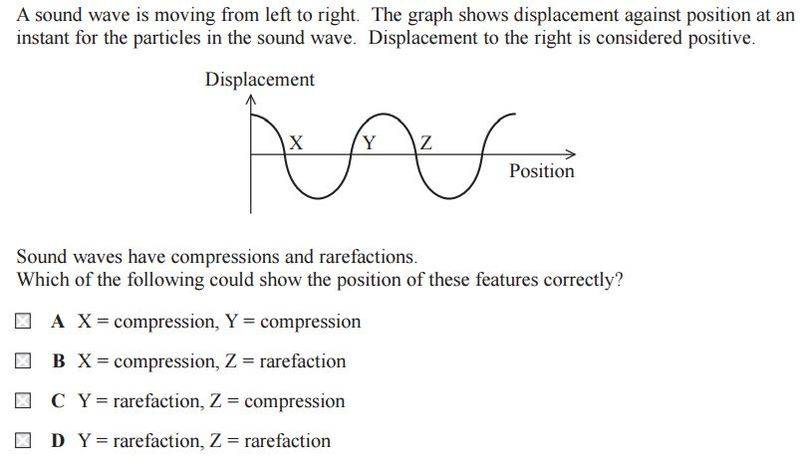# Compressions and rarefactions of a wave

## Homework Statement## The Attempt at a Solution

[/B]
Slightly confused here.

Since this is a displacement-time graph, is it correct to say that at X, since the displacement is zero that it can't be a point of compression because that would imply the air molecules are moving to and fro so they would be experiencing some displacement?

If so, then all three points would be points of rarefaction (since at rarefaction the air molecules aren't moving thus displacement = 0) so the answer would be D?

Simon Bridge
Homework Helper
Since this is a displacement-time graph, is it correct to say that at X, since the displacement is zero that it can't be a point of compression because that would imply the air molecules are moving to and fro so they would be experiencing some displacement?

If so, then all three points would be points of rarefaction (since at rarefaction the air molecules aren't moving thus displacement = 0) so the answer would be D?
Rarefaction means a place where the molecules are spread out.
x is the position the molecule was, y is the current displacement from that position.
So max displacement, either direction, is at a place with no molecules in it... so is that a compression or a rarefaction?

•ravsterphysics
As to your answer that displacement at X is zero, it is correct. But the displacements at Y and Z are also zero.
How can this be?
The reason is that both compressions and as well as rarefactions are corresponding to zero displacements. When you say the displacement is zero, the distance from the current position of the particle to its initial position is always considered. So it is only in compressions and rarefactions, that the said distance is zero.
Then we have to find whether the points X,Y and Z are compressions or rarefactions.
The initiating point of the graph can be considered as a point with maximum displacement. We know that the motionsum of these particles are harmonic motions.So once a particle undergoes maximum displacement, it has no option but to move back towards the centre of oscillation, that is the initial position. So when particles get closer to the centre of oscillation, the pressure will increase ( because particles are more closer to each other). An increase in pressure is a compression. So point X indicates a compression.
Once again after X, a negative peak is obtained. That peak is obtained for a negative displacement.That is a displacement towards the opposite directions. After undergoing negative displacement, the particles move further away from each other, in order to come back to the centre of oscillation. Therefore point Y should be a rarefaction because when particles move away from each other the pressure drops. Then afterwards position Z = X. So point Z is also a compression.

•ravsterphysics
Simon Bridge
Homework Helper
Put another way: consider 5 particles A B C D E F that form part of a longitudinal wave.

The table shows you each partcle, it's equilibrium position, and it's displacement at a particular instance of time:
A 0 0.9
B 1 0
C -2 0.9
D 3 0
E 4 0.9
F 5 0

so, at that time, the particles are at positions:
x = 0.9, 1, 1.1, 3, 3.9, 4, and 4.1 respectively.

Sketch those out on a number line and circle the compressions and the rarifactions.
Spot thier relationship with the displacements.

•ravsterphysics